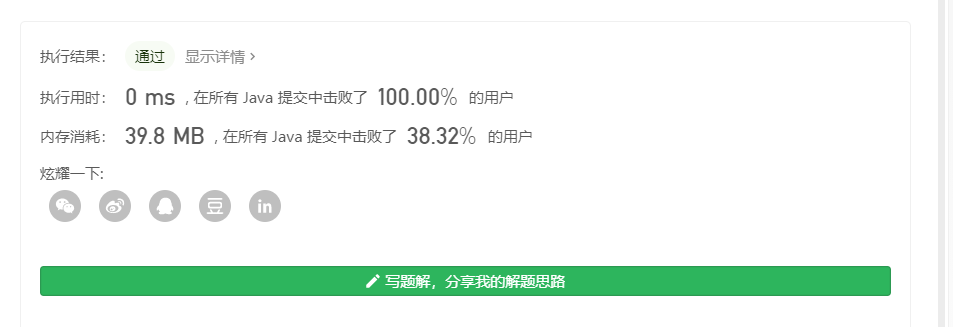### 1.删除链表的倒N结点

public ListNode removeNthFromEnd(ListNode head, int n) {
ListNode dummy = new ListNode(0);
ListNode first = dummy;
ListNode second = dummy;
for (int i = 1; i <= n + 1; i++) {
first = first.next;
}
while (first != null) {
first = first.next;
second = second.next;
}
second.next = second.next.next;
return dummy.next;
}


/**
* public class ListNode {
*     int val;
*     ListNode next;
*     ListNode(int x) { val = x; }
* }
*/
class Solution {
public ListNode removeNthFromEnd(ListNode head, int n) {
ListNode dummy = new ListNode(0);
ListNode first = dummy, second = dummy;
while(n >= 1){
if(first == null)   return dummy.next;
first = first.next;
n--;
}
while(first.next != null){
first = first.next;
second = second.next;
}
second.next = second.next.next;
return dummy.next;
}
}


### 2.返回链表的中间结点

/**
* public class ListNode {
*     int val;
*     ListNode next;
*     ListNode(int x) { val = x; }
* }
*/
class Solution {
while(fast != null && fast.next != null){
slow = slow.next;
fast = fast.next.next;
}
return slow;
}
}


### 3.单链表反转

/**
* public class ListNode {
*     int val;
*     ListNode next;
*     ListNode(int x) { val = x; }
* }
*/
class Solution {
ListNode prev = null;
ListNode next;
while(curr != null){
next = curr.next;
curr.next = prev;
prev = curr;
curr = next;
}
return prev;
}
}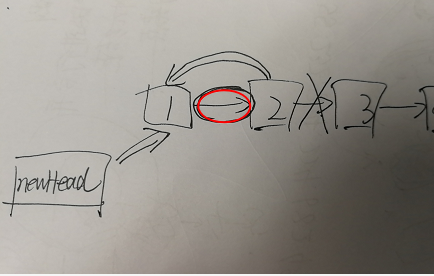/**
* public class ListNode {
*     int val;
*     ListNode next;
*     ListNode(int x) { val = x; }
* }
*/
class Solution {
ListNode p = null;
while(temp != null){
p = temp.next;
temp = p;
}
}
}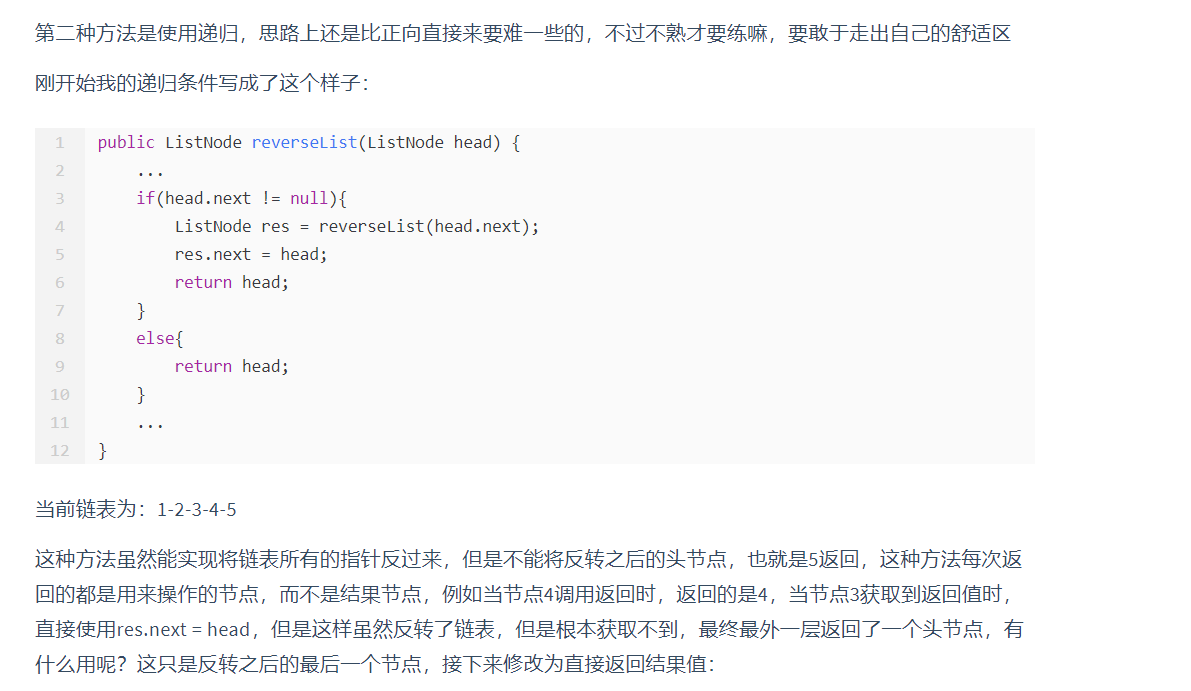/**
* public class ListNode {
*     int val;
*     ListNode next;
*     ListNode(int x) { val = x; }
* }
*/
class Solution {
private ListNode newHead = new ListNode(0);
//为了良好的编程习惯，在程序完成之后需要检查是否有可以合并的条件，例如下面这样，将判断条件和递归终止条件直接合为一个，棒~~~
return res;
}
}


### 4.链表中是否有环

/**
* class ListNode {
*     int val;
*     ListNode next;
*     ListNode(int x) {
*         val = x;
*         next = null;
*     }
* }
*/
public class Solution {
HashSet<ListNode> set = new HashSet<ListNode>();
if(flag == false)   return true;
}
return false;
}
}


/**
* class ListNode {
*     int val;
*     ListNode next;
*     ListNode(int x) {
*         val = x;
*         next = null;
*     }
* }
*/
public class Solution {
while(fast != null && fast.next != null){
fast = fast.next.next;
slow = slow.next;
if(fast == slow)    break;
}
while(fast != null && fast.next != null){
if(fast == slow)    return slow;
slow = slow.next;
fast = fast.next;
}
return null;
}
}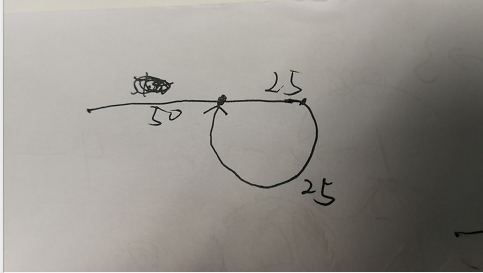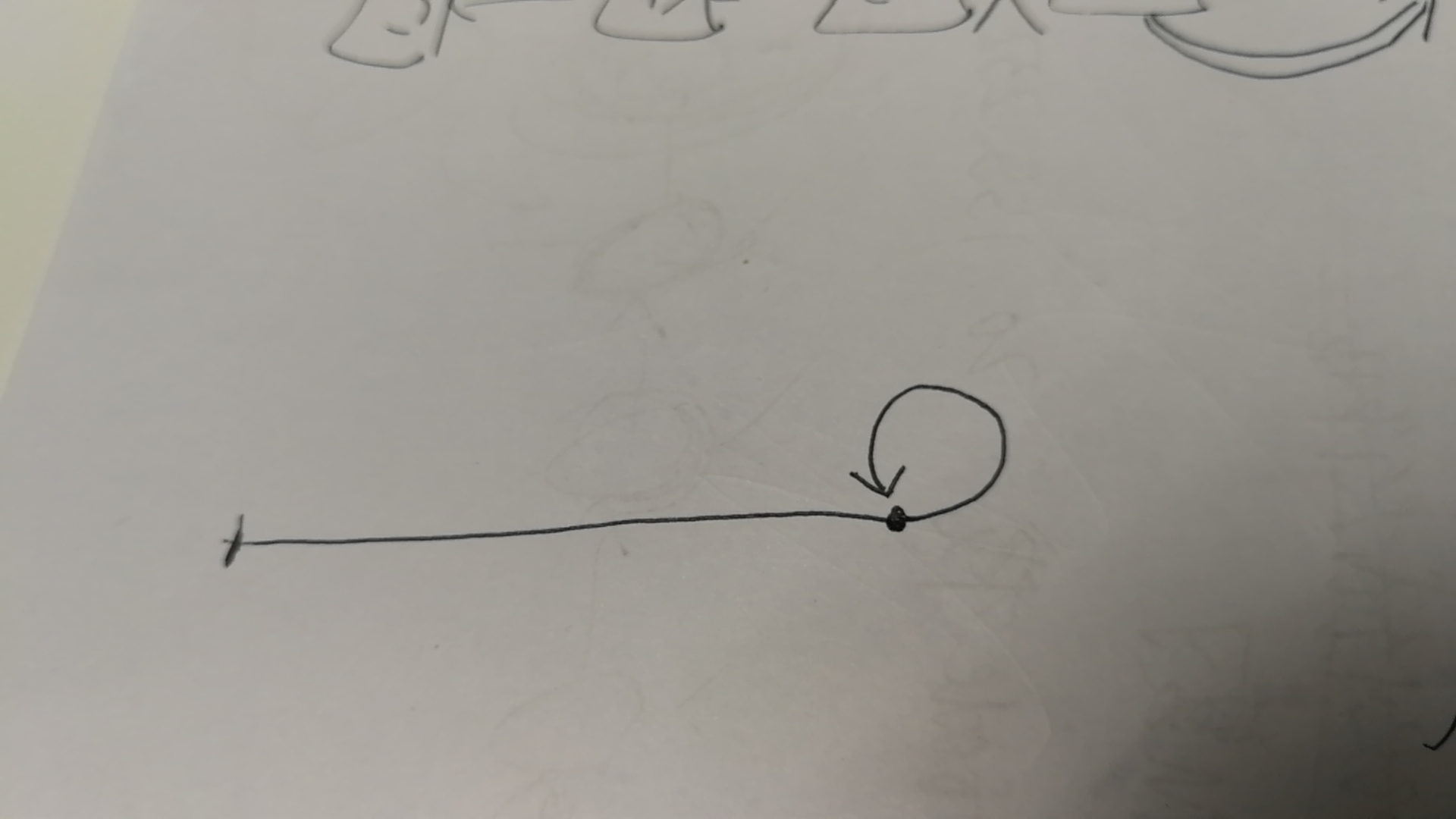### 5.两个有序链表进行合并

class Solution {
public ListNode mergeTwoLists(ListNode l1, ListNode l2) {
ListNode dummy = new ListNode(0);
ListNode temp = dummy;
while(l1 != null && l2 != null){
if(l1.val <= l2.val){
temp.next = l1;
l1 = l1.next;
}else{
temp.next = l2;
l2 = l2.next;
}
temp = temp.next;
}
// if(l2 == null){
//     temp.next = l1;
// }
//   if(l1 == null){
//     temp.next = l2;
// }
//代码整洁之道
temp.next = l1 == null ? l2 : l1;
return dummy.next;
}
}


/**
* public class ListNode {
*     int val;
*     ListNode next;
*     ListNode() {}
*     ListNode(int val) { this.val = val; }
*     ListNode(int val, ListNode next) { this.val = val; this.next = next; }
* }
*/
class Solution {
public ListNode mergeTwoLists(ListNode l1, ListNode l2) {
if(l1 == null)  return l2;
else if(l2 == null) return l1;
else if(l1.val <= l2.val){
l1.next = mergeTwoLists(l1.next,l2);
return l1;
}
else{
l2.next = mergeTwoLists(l1,l2.next);
return l2;
}

}
}